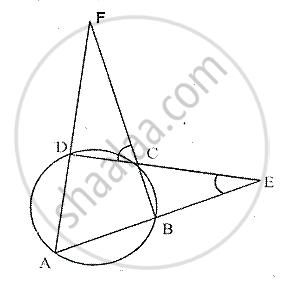Share

# Abcd is a Cyclic Quadrilateral. Sides Ab and Dc Produced Meet at Point E; Whereas Sides Bc and Ad Produced Meet at Point F. If ∠Dcf : ∠F : ∠E = 3 : 5 : 4, Find the Angles of the Cyclic Quadrilate - Mathematics

Course

#### Question

ABCD is a cyclic quadrilateral. Sides AB and DC produced meet at point E; whereas sides BC and AD produced meet at point F.   If ∠DCF : ∠F : ∠E = 3 : 5 : 4, Find the angles of the cyclic quadrilateral ABCD.

#### SolutionGiven – In a circle, ABCD is a cyclic quadrilateral AB and DC
Are produced to meet at E and BC and AD are produced to meet at F.
∠DCF : ∠F : ∠E = 3 : 5 : 4
Let ∠DCF = 3X,∠F = 5x,∠E = 4x
Now, we have to find, ∠A,∠B,∠C and ∠D
In cyclic quad. ABCD, BC is produced.
∴ ∠A =∠DCF = 3x
In ΔCDF,
Ext ∠CDA =∠DCF +∠ 3x +5x = 8x
In ΔBCE,
Ext ∠ABC =∠BCE +∠E       [∠BCE =∠DCF, Vertically opposite angles]
= ∠DCF +∠E

= 3x + 4x = 7x
Since, ∠B + ∠ = 180°
[since sum of opposite of a cyclic quadrilateral are supplementary]
⇒ 7x + 8x = 180°
⇒ 15x = 180°

⇒ x = (180°)/15 = 12°
∠A = 3x = 3 × 12° = 36°
∠B = 7x = 7×12° = 84°
∠C = 180° - ∠A = 180° - 36° = 144°
∠D = 8x = 8 × 12° = 96°

Is there an error in this question or solution?